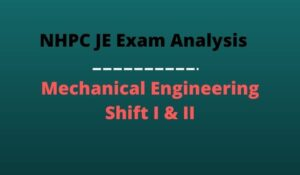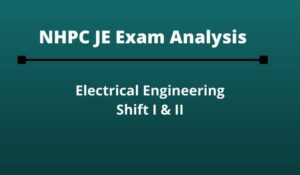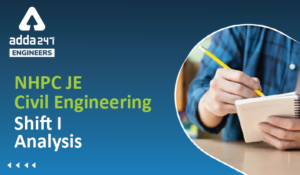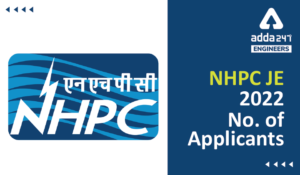Engineering Jobs   »   Civil Engineering quizs   »   NHPC-JE

# NHPC-JE’21 CE: Daily Practices Quiz. 21-Sep-2021

Know your strengths and practice your concepts with this quiz on NHPC JE Recruitment 2021. This quiz for NHPC JE Recruitment 2021 is designed specially according to NHPC Syllabus 2021.

Quiz: Civil Engineering
Exam: NHPC-JE
Topic: Miscellaneous

Each question carries 1 mark
Negative marking: 1/4 mark
Time: 8 Minutes

Q1. Oil is flowing in a pipe of 30 cm diameter with a velocity of 2m/s. at another section of the pipe, the diameter is 20 cm. what will be the velocity of flow at that section?
(a) 3 m/s
(b) 7.5 m/s
(c) 4.5 m/s
(d) 6 m/s

Q2. The shear stress at a point in a shaft subjected to a torque is
(a) Directly proportional to the polar moment of inertia and to the distance of the point form the axis
(b) Directly proportional to the applied torque and inversely proportional to the polar moment of inertia
(c) Directly proportional to the applied torque and the polar moment of inertia
(d) Inversely proportional to the applied torque and the polar moment of inertia

Q3. Coefficient of earth pressure at rest is given by
(a) μ²/(1-μ²)
(b) μ/(1-μ)
(c) (1-μ)/μ
(d) (1–μ²)/μ²

Q4. If the duty of a crop is 864 hectare/cumec and the base period is 120 days, then what is the delta of the crop?
(a) 60 cm
(b) 120 cm
(c) 100 cm
(d) 90 cm

Q5. What is the mechanical widening required for a pavement of width 7m on a horizontal curve of radius 490m, if the longest wheel base of vehicle expected on the road is 7.0m?
(a) 0.07 m
(b) 0.05 m
(c) 0.49m
(d) 0.1m

Q6. Shear reinforcement in a RCC beam is provided to resist
(a) Diagonal tension
(b) Bending stress
(c) Compressive stress
(d) Punching shear

Solutions

S1. Ans.(c)
Sol. From theorem of continuity
A_1 V_1=A_2 V_2
π/4 d_1^2.V_1= π/4 d_2^2.V_2
d_1^2.V_1=d_2^2.V_2
(0.30)^2.2=(0.20)^2 XY_2
4.5m\/ sec⁡〖= V_2 〗

S2. Ans.(b)
Sol. By torsion equation –
T/J=τ/R=Gθ/L
Torque (τ) x Applied torsion (T)
τ α 1/J
J= Polar moment of Inertia

S3. Ans.(b)
Sol. Coefficient of earth pressure at rest (K_o )
▭(K_o=μ/(1-μ))
For sand ▭(K_o=1-sinϕ)

S4. Ans.(b)
Sol. Given
Duty = 864 hectare/cumec
Base period = 120 days
∆= ?
Relation between duty and delta is, ∆ = 8.64 B/D
= 8.64×120/864
= 1.2 m.or 120 cm

S5. Ans.(d)
Sol. Given, length of wheel base (l) = 7m
Radius (R) = 490m.
No. of lanes (n) = 2
mechanical widening W_me = (nl^2)/2R
= (2×(7)^2)/(2×490) = 0.1

S6. Ans.(a)
Sol. Shear reinforcement in RCC beam is provided to resist diagonal tension. Stirrups is provided in the beam for shear reinforcement. At corner spacing between stirrups decreases and increase towards center.

Sharing is caring!

Thank You, Your details have been submitted we will get back to you.
•NHPC Result Through GATE 2021, Download ...
•NHPC JE Mechanical Exam Analysis 2022 Di...
•NHPC JE Exam Analysis 2022 Electrical En...
•NHPC JE Exam Analysis Civil Engineering ...
•NHPC JE Application Form 2022, Check No....
•NHPC Junior Engineer Admit Card 2022, Di...